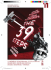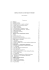# 8.4 Dividing Integers

## Transcription

8.4 Dividing Integers
```Dividing Integers
Focus on…
After this lesson, you
will be able to...
φ determine integer
φ
quotients using a
number line
apply a sign rule
when dividing
integers
Farmers around the world use fertilizers made from potash mined in
Saskatchewan. The province produces over 40% of the world’s supply
of potash.
To reach the potash, miners are lowered down a vertical mineshaft in a
cage. Typical mineshafts are 900 m to 1100 m deep. The cage descends
at about 6 m/s. How could you use integer chips to determine the time it
takes to descend 900 m? Describe any difficulty you see in using integer
chips to determine the time.
How can you divide two integers?
• red and blue integer
chips
1.
The diagram shows how you can model the division (+15) ÷ (+3)
using a number line.
−1
0
+1
+2
+3
+4
+5
+6
+7
+8
+ 9 + 10 + 11 + 12 + 13 + 14 + 15
How are the two integers in the division (+15) ÷ (+3) shown
in the diagram?
b) Model (+15) ÷ (+3) using integer chips. What is the quotient?
c) How does the number line show the quotient?
d) Explain how the diagram can also model the division
(+15) ÷ (+5).
a)
306
MHR • Chapter 8
08_ML8_Chapter08_6th.indd 306
4/9/08 4:24:18 PM
2.
The diagram shows how you can model the division (-15) ÷ (-3)
using a number line.
−16 −15 −14 −13 −12 −11 −10 −9
−8
−7
−6
−5
−4
−3
−2
−1
0
+1
How are the two integers in the division (-15) ÷ (-3) shown
in the diagram?
b) Model (-15) ÷ (-3) using integer chips. What is the quotient?
c) How does the number line show the quotient?
d) Explain how the diagram can also model the division
(-15) ÷ (+5).
a)
Model the division (-15) ÷ (+3) using a number line. Explain
b) Copy and complete the division statement (-15) ÷ (+3) = .
c) Explain how your diagram can also model the division
(-15) ÷ (-5).
3. a)
4.
Can you use the same methods as in #1 to #3 to model the division
(+15) ÷ (-3)? Explain.
5.
The first row of the table shows a multiplication statement and the
two division statements related to it. Copy and complete the table.
Multiplication Statement
(+2) × (+4) = +8
Related Division Statements
(+8) ÷ (+4) = +2
(+8) ÷ (+2) = +4
(+6) × (+2) = +12
(+3) × (-5) = -15
(-3) × (+6) = -18
(-5) × (-4) = +20
(-1) × (-9) = +9
6.
Copy each of the following statements. Use your results from the
table to complete each statement using the word “positive” or the
word “negative.”
The quotient of two integers with the same sign is .
The quotient of two integers with different signs is .
How can you use a number line to divide two integers? In your
description, state any limitations of your method.
b) How can you use the signs of two integers to help determine
their quotient?
7. a)
8.4 Dividing Integers • MHR
08_ML8_Chapter08_6th.indd 307
307
4/9/08 4:24:21 PM
Example 1: Divide Integers
Calculate.
a)
c)
sign rule
(for division)
• the quotient of two
integers with the same
sign is positive
• the quotient of two
integers with different
signs is negative
a)
c)
d)
308
b)
d)
(-12) ÷ (-6)
(+42) ÷ (-14)
Solution
Divide the numerals and then apply a sign rule.
b)
To enter a positive
integer on your
calculator, you do not
need to enter the
positive sign. You do
need to enter the
negative sign for a
negative integer. On
most calculators, the
key used to enter a
negative sign is not
the subtraction key.
Check that the key
sequence shown in
Example 1d) works
correctly on your
calculator. Modify
the sequence, if
necessary.
(+6) ÷ (+2)
(-20) ÷ (+4)
6÷2=3
The integers +6 and +2 have
the same sign, so the quotient
is positive.
(+6) ÷ (+2) = +3
12 ÷ 6 = 2
The integers -12 and -6
have the same sign, so the
quotient is positive.
(-12) ÷ (-6) = +2
20 ÷ 4 = 5
The integers -20 and +4
have different signs, so the
quotient is negative.
(-20) ÷ (+4) = -5
You can also determine the
quotient using a number line.
0
+1
−14 −12 −10 −8
+2
−6
+3
−4
+4
−2
+5
+6
0
+2
−20 −18 −16 −14 −12 −10 −8 −6 −4 −2 0 +2
42 ÷ 14 = 3
The integers +42 and -14 have different signs, so the quotient is
negative.
(+42) ÷ (-14) = -3
C 42 ÷ 14 + - = -3.
Check:
(-3) × (-14) = +42
Calculate.
a) (+24) ÷ (+8)
c) (-48) ÷ (-12)
You can use multiplication to check
(+30) ÷ (-10)
d) (-66) ÷ (+11)
b)
MHR • Chapter 8
08_ML8_Chapter08_6th.indd 308
4/9/08 4:24:21 PM
Example 2: Apply Integer Division
Daria and four of her friends went out for lunch. They agreed to split
the cost equally. The total bill came to \$85, which Daria paid on her
credit card. How much did each of her friends owe Daria?
Solution
Use the division of two integers to represent the situation.
Represent the total cost of \$85 by the integer -85.
Represent the 5 people by the integer +5.
Each person’s share can be represented by the expression (-85) ÷ (+5).
(-85) ÷ (+5) = -17
Each of her friends owed Daria \$17.
85 ÷ 5 = 17. The
integers -85 and +5
have different signs, so
the quotient is negative.
Check.
Use multiplication to check the division.
(-17) × (+5) = -85
Pierre paid \$42 to admit himself and two of his friends into a science
museum. What was the cost of each admission?
• You can model some integer divisions on a number line.
−12 −11 −10 −9
−8
−7
−6
−5
−4
−3
−2
−1
0
+1
(-12) ÷ (-4) = +3
(-12) ÷ (+3) = -4
• You can divide two integers by dividing the numerals and applying the sign rules.
The quotient of two integers with the same sign is positive.
(+6) ÷ (+2) = +3
(-6) ÷ (-2) = +3
The quotient of two integers with different signs is negative.
(+6) ÷ (-2) = -3
(-6) ÷ (+2) = -3
1.
To model the division (+15) ÷ (+3) on a number line, you first draw
an arrow that represents +15. You then have two choices:
• You can cut the arrow into parts that each represent +3 and count
how many parts there are.
• You can cut the arrow into three equal parts and determine the value
that each part represents.
Which choice do you prefer? Explain.
8.4 Dividing Integers • MHR
08_ML8_Chapter08_6th.indd 309
309
4/9/08 4:24:23 PM
2.
Aziza used a number line to model the division (-12) ÷ (-2). Yuri used
a number line to model the division (-12) ÷ (+6). They drew the same
diagram. What was the diagram?
3.
Michel said, “When I divide +6 by +3, +2, or +1, the quotient is less than
or equal to +6. If I divide -6 by +3, +2, or +1, I think the quotient should
be less than or equal to -6.” Do you agree with him? Explain.
4.
Without doing any calculations, Stefani said that the quotients
(-252) ÷ (-18) and (+252) ÷ (+18) must be the same. How did she know?
9.
For help with #5 to #10, refer to Example 1 on
page 308.
5.
Write two division statements that each
diagram could represent.
10.
a)
+4
+6
+8 +10 +12 +14 +16 +18
−12 −10 −8
−6
−4
0
+2
Calculate and check.
a) (+20) ÷ (+5)
c) (-57) ÷ (+19)
b)
Calculate.
a) (-26) ÷ (-26)
c) 0 ÷ (-33)
b)
(+36) ÷ (-6)
d) (-84) ÷ (-42)
(+95) ÷ (-5)
d) (-68) ÷ (+17)
b)
6.
−2
0
+2
Write two division statements that each
diagram could represent.
For help with #11 to #15, refer to Example 2 on
page 309. Use the division of two integers to
represent each situation and solve the problem.
a)
11.
−10 −8
−6
−4
−2
0
+2
+4
+2
+4
+6
+8 +10 +12 +14 +16
b)
−2
7.
8.
310
0
Determine each quotient using a number line.
a) (+12) ÷ (+6)
b) (-20) ÷ (-4)
c) (-8) ÷ (+4)
d) (-10) ÷ (-5)
Determine each quotient using a number line.
a) (-14) ÷ (-7)
b) (+16) ÷ (+4)
c) (-22) ÷ (+2)
d) (-15) ÷ (-5)
Raoul borrowed \$15 per month from his
mother to pay for the art supplies he
needed for an evening class. At the end of
the course, he owed his mother \$60. How
long was the course?
A submarine took 16 min to dive 96 m
from the surface. How far did it dive
per minute?
b) The submarine took 12 min to climb
back to the surface. How far did it
climb per minute?
12. a)
MHR • Chapter 8
08_ML8_Chapter08_6th.indd 310
4/9/08 4:24:23 PM
13.
14.
A scuba diver was collecting water samples
from a lake. He collected samples at 5-m
intervals starting at 5 m below the surface.
He collected the final sample at a depth of
35 m. How many samples did he collect?
Mina was drilling down through a 21-cm
thick concrete floor to install a new
plumbing pipe. She drilled for 5 min, took a
break, and then finished drilling in another
2 min. At what rate did the drill cut through
the floor, in centimetres per minute? What
assumptions did you make?
15.
A school spent \$384 to buy a set of
32 calculators. What was the cost of
each calculator?
16.
Without evaluating the quotients, identify
the quotient with the least value. Explain
(+2408) ÷ (+43)
(-2408) ÷ (-43)
(+2408) ÷ (-43)
17.
If 28 times an integer is -448, what is
the integer?
18.
Copy and complete each statement.
a) (+72) ÷ () = +9
b) () ÷ (+12) = -10
c) () ÷ (-13) = -11
d) (-84) ÷ () = +6
19.
Write a word problem that you can solve
using the expression (-80) ÷ (+16).
20.
Create your own word problem that
involves integer division. Make sure that
you can solve your problem and that the
calculation results in an integer. Give your
problem to a classmate to solve.
21.
Describe each pattern. Then write the
next three terms in each pattern.
a) +125 000, +25 000, +5000, +1000, …
b) -512, +256, -128, +64, …
c) -1 000 000, -100 000, -10 000,
-1000, …
d) +1458, -486, +162, -54, …
22.
The sum of two integers is +20. Dividing
the larger integer by the smaller integer
gives a quotient of -3. What are the
two integers?
The temperature of still, dry air decreases by about 6 °C for each kilometre increase in
altitude. On a still, dry day, the temperature in Yellowknife, Northwest Territories,
was -11 °C . The air temperature outside a plane flying above Yellowknife was -53 °C.
a) Approximately how much lower was the temperature outside the aircraft than the
temperature in Yellowknife?
b) How high was the aircraft above Yellowknife?
8.4 Dividing Integers • MHR
08_ML8_Chapter08_6th.indd 311
311
4/9/08 4:24:24 PM
```

### Managers` Towering Personality with 14Qs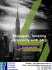### Problem Set### 17) Summa Brasiliensis Mathematicae, II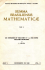### D I V I S I O N ...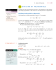### Chapter 8 - SchoolRack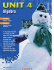### Pre-Algebra With Pizzazz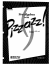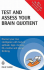### Divide Whole Numbers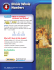### File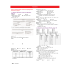### Divide by a Two-Digit Divisor - Macmillan/McGraw-Hill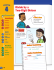### Unparalleled built into every home.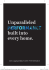### examensarbeten i matematik - Matematiska institutionen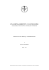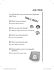### TestAS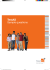### fall activities - We Support Our Local Schools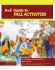### Treatment Card_RZ.indd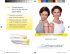### Numeration 2016 - Katedra matematiky### Lifetouch Yearbooks®. We`ll make it easy, you`ll make it great.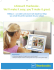### tsNN 0002 .. 1`148 AGSOA 10 V 17 (D) 1 à B0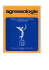### You can our programme by clicking